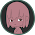Monday, June 16, 2014

The Solution to My Gain Problem - Goofy Op Amps

If you remember,
http://jazz-disassemblies.blogspot.com/2014/06/toying-with-gain-peek-at-differential.html
I had a problem with an operational amplifier. My circuit for multiplying by a fractional gain was successful. 1/2 gain, 1/3 gain, no problem. But when I wanted to increase gain by 2, the output voltage was much too high; exactly +5v too high in all cases. The problem was how to reduce my output by 5v in all cases, but how?

The op amp circuit that we used was a differential amplifier, which means the difference between two input voltages is amplified, and not any one voltage input. I finally found this web site with a much simpler equation:
http://electronics.stackexchange.com/questions/94043/op-amp-design-resistor-values-vs-actual-values-gain-calculation

The equation, Vout = -(R2/R1)(V1-V2)
I knew that the gain was determined by R2/R1
and that the Vin would be 0 to 5v
and Vout should be -5v to +5v

Since the gain I wanted was 2 and I knew my in and out signals, I needed to find V1, which would become my reference voltage. I thought my reference would be 0, because I want my output signal centered at 0v BUT, it is not in fact the center point of our output. It turns out that the reference voltage is also multiplied by the gain and then added to the output. If my output is 5v too high in all cases, then we need to add (-5v/2).
Therefore my range would be:

-(gain)*(V1-V2) = Vout
so,
(-2) * (2.5-5) = -2*-2.5 = +5v
and,
(-2) * (2.5-0) = -2*2.5 = -5v

There is my range! And there is my reference voltage!

R2 = 100K ohms
R1 = 50K ohms
Vref = 2.5v
so,
Gain = 2
Vin = 0/5v
Vout = -5/+5v (10vpp)

If I connect my supply voltages to +5v and -5v, then any voltages outside of that range will be clipped off, protecting any other devices this may be connected to along the line. Success!

1 comment:

1.I mentioned the excess voltage being clipped off, but in reality it will actually be mirrored. This is called phase reversal and will be found in most op amps, including the TL072 which I like very much. To actually see voltage outside of our range clipped, we need to locate an op amp that specifies clipping in the datasheet. The MCP6002 is one such op amp.

Cheers,
Jordan